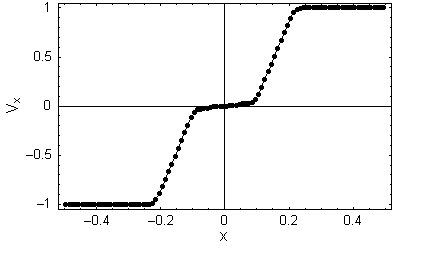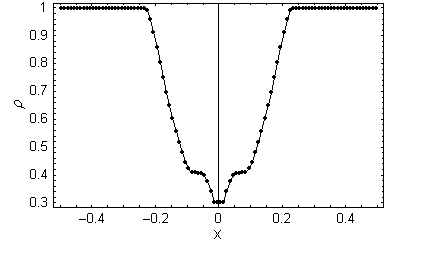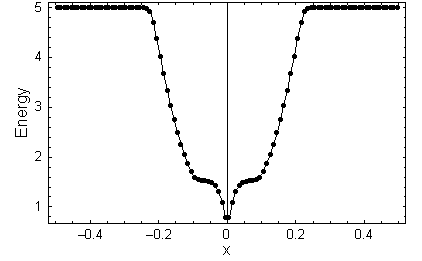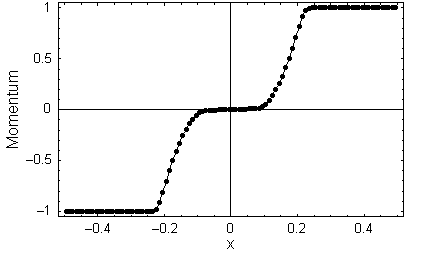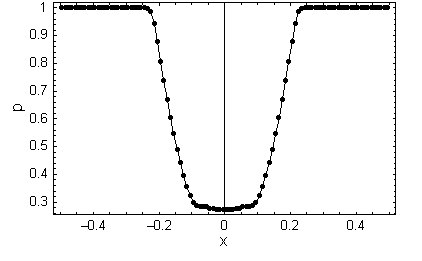# Einfeldt's Strong Rarefaction Tests

## Reference:

Einfeldt, et al., "On Godunov-Type Methods near Low Densities.", J. Computational Physics, 92, 273-295 (1991). The computational tests are descrived in Section 6.

## Description:

These tests are Riemann problems where the left and right states are described for the first test (1-2-0-3) as follows. Left: (ρ=1; m=-2; n=0;e=3) Right: (ρ=1,m=2;n=0; e=3), where m = ρu ex and n = ρv ey are the momentum densities, and u and v are the velocities in the x and y directions, respectively. These initial conditions launch two rarefactions wave, one moving to the right and one moving to the left. The other two initial conditions adopt this notation for the inital states as (1-1-0-5) and (1-1-2-5). This latter test introduces a shear flow perpendicular to the rarefactions.

Linearized Riemann solvers can fail by returning negative pressures or densities in one or more of the intermediate states for very strong rarefactions. The series of tests introduced by Einfeldt are designed to demonstrate this failure mode. The paper shows that HLLE-schemes guarantee positivity when they satisfy certain constraints. In Athena, we use the HLLE solver whenever the Roe solver returns a negative pressure or density in an intermediate state. The test results below demonstrate how well this procedure works.

## Results:

Results computed with Athena using the third-order Roe solver on an array of 100 cells with a Courant number of 0.8 and γ = 1.4. The velocity, Mach number, density, energy, momentum, and pressure are plotted for each of the three tests. Test (1-2-0-3) may be compared to Figs. 6.1-6.3 in the reference. Test (1-1-0-5) may be compared to Fig. 6.4 and test (1-1-2-5) may be compared to Figs. 6.5-6.6. The HLLE solver was required for only one interface, and for one timestep (the first) in the 1-2-0-3 test; thereafter the Roe solver worked fine.

## Test (1-2-0-3)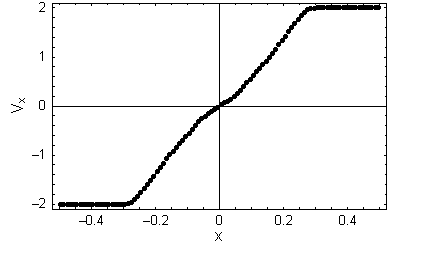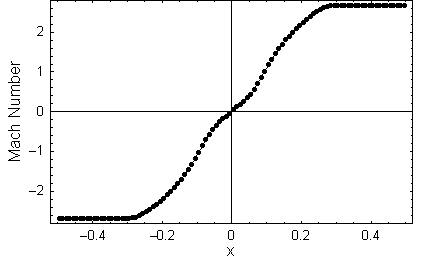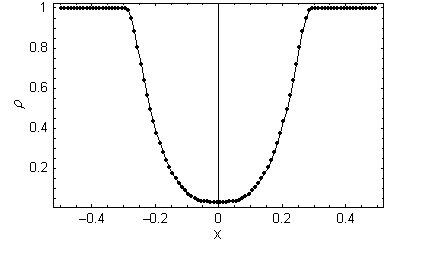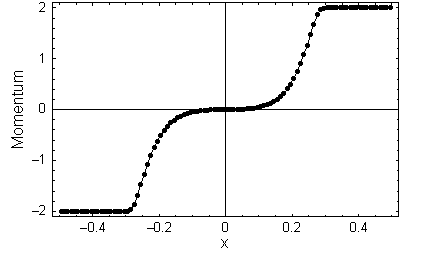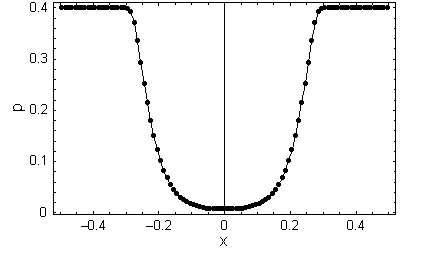## Test (1-1-0-5)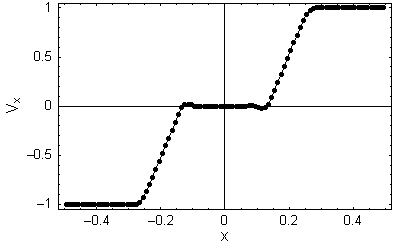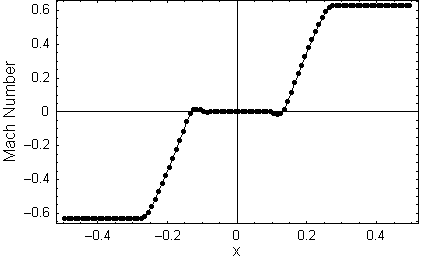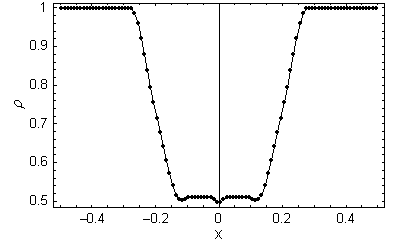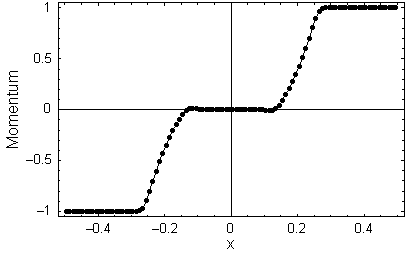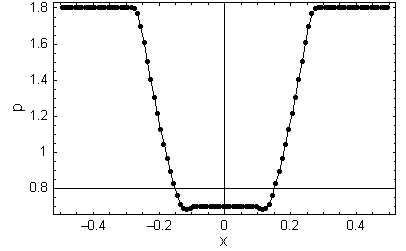## Test (1-1-2-5)